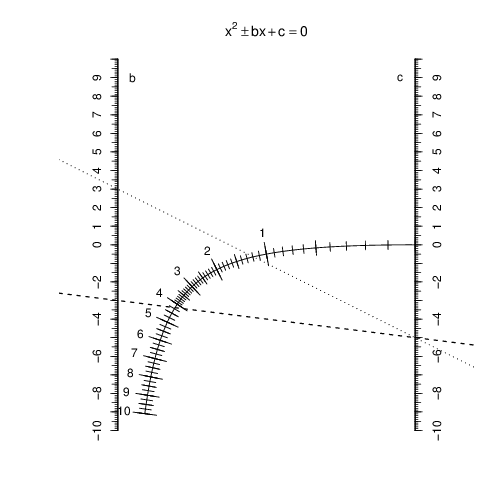## Intro

[This is a page from Jonathan Dushoff’s math wiki, adapted for use as a test of my Working Markup blog-post system. Scroll down for info about how this post is made, including source code. -lw]

On this page, I use the free software environment R to construct a nomogram that I read about in a recreational math book when I was a kid. This nomogram solves quadratic equations.

## Nomogram

You can print out the nomogram, and use a ruler to read off the solutions to the quadratic equation, where the line from $\pm b$ to $c$ intersects the scale.The example above shows the solutions to the equation $x^{2}-3x-5=0$. The dashed line connecting $b$ to $c$ shows the positive solution (4.2), and the dotted line connecting $-b$ to $c$ shows the negative solution (-1.2).In this example ($x^{2}-5x+1=0$), both solutions are positive (0.2 and 4.8), thus only the dashed line intersects the scale.

## Math

We want to draw lines from $(0,b)$ to $(1,c)$ that will pass through a point labeled with $r$, where $r$ is a root, satisfying $r^{2}+br+c=0$. The equation for our line is $y=b+(c-b)x$. Solve the quadratic to obtain $c=-r^{2}-br$, and substitute into the line equation to get $y=b-(r^{2}+br+b)x$.

If we want all lines corresponding to $r$ to go through our point, we must find one independent of $b$. We do this by setting $x=1/(r+1)$, to yield $y=-r^{2}/(r+1)$.

## Code

Define and call a nomogram function.

nomogram.R
dev.off()
pdf(height=9.5, width=6.5)
nomogram <- function(xoff=0.1, asp=1/16){
plot(NULL, NULL, asp=asp,
main = expression(x**2 %+-% bx + c == 0),
xlim=c(-xoff, 1+xoff), ylim=c(-10,10), axes=FALSE,
xaxs="i", yaxs="i",
xlab="", ylab=""
)

vertical_axis <- function(pos, side=2){
axis(side, pos=pos, at=-10:10)
axis(side, pos=pos, at= seq(-10, 10, by=0.5),
labels=FALSE, tcl=-0.4)
axis(side, pos=pos, at= seq(-10, 10, by=0.1),
labels=FALSE, tcl=-0.2)
}

vertical_axis(0)
vertical_axis(1, 4)
text(0.05, 9, "b")
text(0.95, 9, "c")

r = seq(0, 10, length.out=1001)
lines(1/(r+1), -r^2/(r+1))

rticks <- function(r, tcl, asp=1, labels=FALSE, ll=0.9){
x <- 1/(r+1)
y <- -r^2/(r+1)
yx <- (r^2+2*r)*asp
x0 <- x+yp*tcl/2
x1 <- x-yp*tcl/2
y0 <- y-xp*tcl/2
y1 <- y+xp*tcl/2
arrows(x0, y0, x1, y1, length=0)
if(labels){
text(x-ll*yp*tcl, y+ll*xp*tcl, r)
}
}

rticks(seq(1, 10, by=1), 0.08, asp, labels=TRUE)
rticks(seq(0, 10, by=0.5), 0.05, asp)
rticks(seq(0, 4, by=0.1), 0.03, asp)
rticks(seq(4, 10, by=0.25), 0.04, asp)
}

nomogram()


Use make to make the example code below depend on the R environment from above (using our custom R rules).

example.mk
example.Rout: nomogram.RData example.R


Make a nomogram, then define and make example lines.

example.R
eqLines <- function(a, b){
abline(a, b-a, lty=2, lwd=1.5)
abline(-a, b+a, lty=3, lwd=1.5)
}
nomogram()
eqLines(-3, -5)


Here’s another example.

another.mk
another.Rout: example.RData


another.R
nomogram()
eqLines(-5, 1)


## Printable

printable.mk
printable.Rout: nomogram.Rout


printable.R
# setpdf(height=9.5, width=6.5) # This is a theobio/Rprep magic command
nomogram()


## Export source code

This project is designed for reproducible research.

• This entire website (including the source text of this blog post, with all the source code included) is available for cloning at https://github.com/worden-lee/github-pages-sandbox. I’m planning to write instructions for processing it soon.
• To reproduce the full blog post you’ll need to have the following installed: Jekyll, git, R, latexml, GNU make, perl (and maybe other things - bug reports welcome).
• Here is an automatically-generated tar file of the source file contents: wmd_export.tgz. You should be able to unpack this directory and simply run ‘make example.Rout.png’ to build the first image above, and similar commands to build the other output files.
• To reproduce these outputs you will need GNU make, perl, and R installed.

To see the source text of this blog post, with the R and makefile code embedded, see https://github.com/worden-lee/github-pages-sandbox/blob/master/_posts/2014-10-15-nomogram.md.wmd.

See also a discussion of how this is put together at http://leeworden.net/lw/node/148 and http://leeworden.net/lw/node/149.